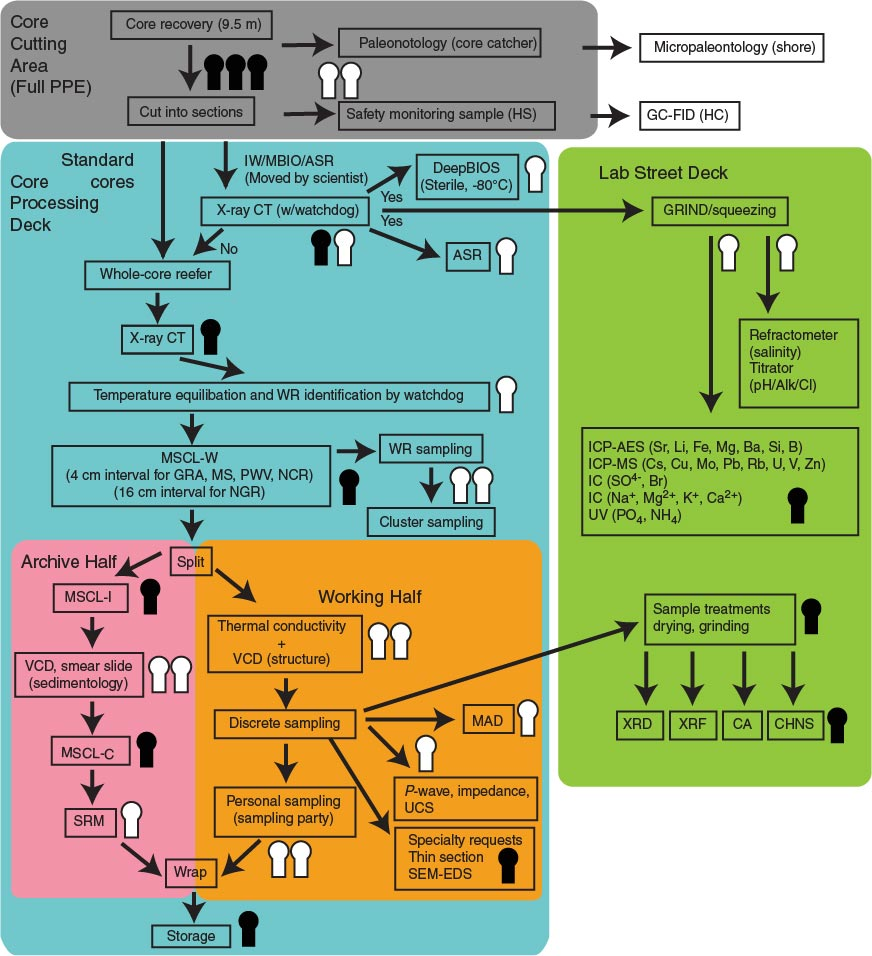Figure F5. Schematic diagram of proposed core flow (based on Expedition 338). PPE = personal protective equipment, HS = headspace, GC-FID = gas chromatograph–flame ionization detector, HC = hydrocarbon, IW = interstitial water, MBIO = microbiological sample, ASR = anelastic strain recovery, DeepBIOS = deep biosphere sample, CT = computed tomography, WR = whole round, MSCL-W = whole-round multisensor core logger, GRA = gamma ray attenuation, MS = magnetic susceptibility, PWV = P-wave velocity, NCR = noncontact resistivity, NGR = natural gamma radiation, MSCL-I = photo image logger, VCD = visual core description, MSCL-C = color spectroscopy logger, SRM = superconducting rock magnetometer, MAD = moisture and density, UCS = unconfined compressive strength, SEM-EDS = scanning electron microscope–energy dispersive spectrometry, GRIND = ground rock interstitial normative determination, ICP-AES = inductively coupled plasma–atomic emission spectroscopy, ICP-MS = inductively coupled plasma–mass spectrometry, IC = ion chromatography, UV = ultraviolet, XRD = X-ray diffraction, XRF = X-ray fluorescence, CA = carbonate analyzer, CHNS = carbon-hydrogen-nitrogen-sulfur analyzer. Black figures = Marine Works Japan Laboratory Technicians, white figures = scientists.